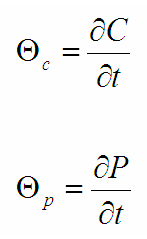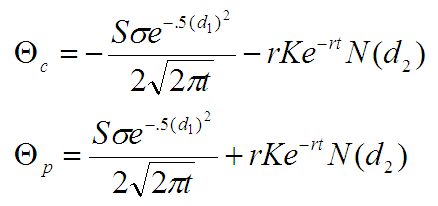# Option Greeks: Theta

Theta is a measure of the sensitivity of an option to the time remaining until expiration. It is a measure of the time decay of the option.

The value of theta is the dollar value that the option will lose each day with the passage of time. Theta value is calculated as a dollar value per year but is converted to a per day value by traders for their portfolios.

It is represented as follows:Theta is greater than zero because more time until expiration means more option value.

Theta is always negative for long calls and puts and positive for short (or written) calls and puts.

The passage of time hurts the option holder while it benefits the option writer. If you are long an option you are short theta, that is your portfolio will lose value with the passage of time (all other factors held constant).

Calculating Theta

For calls and puts, theta is calculated as follows:The equations determine theta as a dollar value per year. A theta of -4.50, for example, means the option will lose \$4.50 in value over a period of one year.

Option Pricing and Greeks Calculator